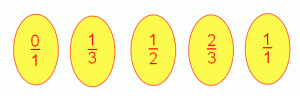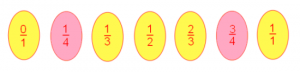It is not difficult to put a list of decimals in order of size. But what about ordering fractions?

John Farey introduced sequences of sequences of fractions in order of size, now called Farey sequences. Can you discover his method from the following examples?The third Farey sequence shown here lists in order,  in their simplest form, all the fractions between 0 and 1 that have denominators 1, 2 and 3.The fourth Farey sequence shown here lists in order, in their simplest form, all the fractions between 0 and 1 which have denominators 1, 2, 3 and 4.

Can you find the fifth Farey sequence? What about sixth Farey sequence and more sequences in this sequence of sequences?

What do you notice about these sequences?